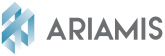# ZERO D, ONE D

## “Zero D” and “One D”, the art of discretising the values of a complex system

### “ZERO D” AND ONE D” CALCULATIONS AND EQUATIONS ARE THE COMPONENTS OF WIDER MODELLING

System modelling calls on a set of calculations and equations known as “Zero D” or “One D”. These different calculations or equations are the variables for a simulation. This parameter segmentation approach makes it possible to simulate the behaviour of smart systems before the detailed geometry is available (Computer Assisted Design). It is further possible to understand the “zero D” or “One D” concept as opposed to three-dimensional representations in space.This approach makes it possible to simulate the behaviour of smart systems before the detailed geometry is available (CAD).### A CALCULATION AND EQUATION LIBRARY TO PREDICT PHYSICAL PHENOMENA AND BEHAVIOURS

The “Zero D” and “One D” concept is used upstream of complex system simulation. Before taking into account physical aspects expressed in 3D, different physical phenomena can be predicted: mechanical, hydraulic, thermal, diphasic, etc.

### MATLAB/SIMULINK, AMESIM AND GT-SUITE, SPECIFIC SYSTEM MODELLING TOOLS THAT USE THE “ZERO D” AND “ONE D” CONCEPT

The various tools are used depending on the progress of the design or its purpose.

MATLAB/SIMULINK is a multidomain dynamic system modelling platform.

AMESIM is simulation software for the modelling and analysis of “One D” multidomain systems (Mechanical, Hydraulic, thermo-hydraulic, hydraulic design, thermal, diphasic, Ifp drive, etc.)

GT-SUITE is “One D” modelling software more specific to the automotive sector

> Fluid flows: cooling, air-conditioning, thermo-management

> Engine thermal engineering, passenger compartment thermal engineering

> Vehicle dynamics

> Injection system

> ...

NATURAL GAS

Natural gas, a credible energy source with a sustainable development focus